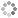# PROWAREtecharticles » current » dot-net » convert-number-to-binary-string

## .NET: Convert Number to Binary String

How to convert a number such as an int, uint, long, ulong or other integer to a binary string using C#.

Just a few lines of code are required to convert a number to a binary string.

``````
public class Convert
{
public static string NumberToBinaryString(ulong bits)
{
string ret = string.Empty;
if(bits == 0)
ret = "0";
else
while (bits != 0)
{
ret += (char)((bits & 1) + '0'); // NOTE: does not use conditional
bits >>= 1;
}
char[] chars = ret.ToCharArray();
Array.Reverse(chars);
return string.Join("", chars);
}
}
``````
###### Two's Complement
``````
string binary = Convert.NumberToBinaryString(4); // binary == "100"
``````
###### Sign Magnitude
``````
int bits = -4;
if(bits < 0)
bits = -bits;
string binary = Convert.NumberToBinaryString((ulong)bits);
``````
###### One's Complement
``````
int bits = -4;
if(bits < 0)
bits--;
string binary = Convert.NumberToBinaryString((ulong)bits);
``````This site uses cookies. Cookies are simple text files stored on the user's computer. They are used for adding features and security to this site. Read the privacy policy.